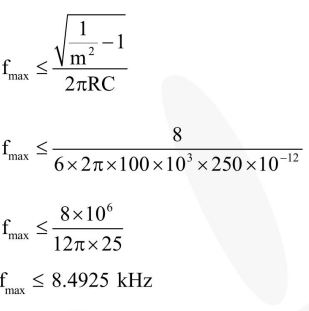# A diode detector is used to detect an amplitude modulated wave of

Question:

A diode detector is used to detect an amplitude modulated wave of $60 \%$ modulation by using a condenser of capacity 250 pico farad in parallel with a load resistance 100 kilo ohm. Find the maximum modulated frequency which could be detected by it.

1. $10.62 \mathrm{kHz}$

2. $5.31 \mathrm{MHz}$

3. $5.31 \mathrm{kHz}$

4. $10.62 \mathrm{MHz}$

Correct Option: , 3

Solution: